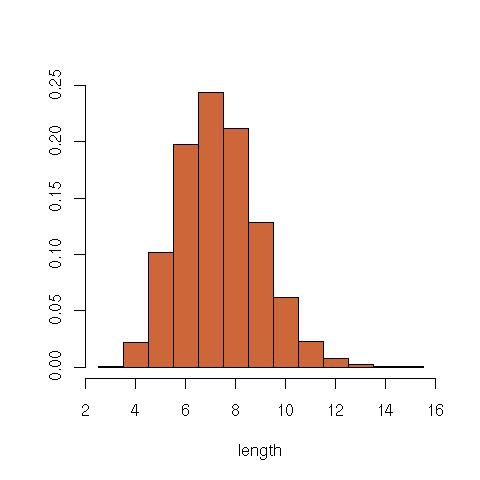Want to share your content on R-bloggers? click here if you have a blog, or here if you don't.

The mathematical puzzle in the latest weekend edition of Le Monde is as follows:

Two kids are given three boxes of chocolates with a total of 32 pieces. Rather than sharing evenly, they play the following game: Each in turn, they pick one of the three boxes, empty its contents in a jar and pick some chocolates from one of the remaining boxes so that no box stays empty. The game ends with the current player’s loss when this is no longer possible. What is the optimal strategy?

This led me to consider a simple branching process starting from a multinomial$(u_1,v_1,w_1)sim mathcal{M}_3(29;1/3,1/3,1/3)$

to define$(x_1=1+u_1,y_1=1+v_1,z_1=1+w_1)$. and then following the above splitting process, namely the selection of the dead and of the split components,$x_t$ and

with the updated value being$(x_{t+1},y_{t+1},z_{t+1}) = (1+u_{t+1},1+v_{t+1},z_t).$

This process is obviously not optimal but on the opposite completely random. Running a short R program like

```N=32
prc=story=rep(1,3)+as.vector(rmultinom(1,(N-3),prob=rep(1,3)))
while (sum(prc)>3){
if (sum(prc>1)==1)
i=(1:3)[prc>1]           #split
else
i=sample((1:3)[prc>1],1) #split
j=sample((1:3)[-i],1)          #unchanged
prc=c(prc[j],1+as.vector(rmultinom(1,prc[i]-2,prob=rep(1,2))))
story=rbind(story,prc)
}```

leads to a histogram of the game duration which is as follows. (Note that the R command sample((1:3)[prc>1]) does not produce what it should when only one term of prc is different from 1, hence the condition.) Obviously, this is not a very interesting branching process in that the sequence always ends up in a few steps…Of course, this does not tell much about the initial puzzle. However, discussing the problem with Antoine Dreyer and Robin Ryder led to Antoine obtaining all winning and loosing configurations up to$N=32$ by a recursive R algorithm and to Robin establishing a complete resolution (I do not want to unveil it before he does!) that involves the funny facts [a] any starting configuration with only odd numbers is loosing and [b] any$N$ that is a power of 2, like 32, always produces winning configurations.

Filed under: R, Statistics Tagged: arithmetics, branching process, Le Monde, mathematical puzzle